## Posts Tagged ‘yamashita keigo’

### Chunlan Cup: Japan is Very Happy After Round One

Saturday, March 27th, 2010

First round of the 8th international Chunlan Cup took place in China. The only non-Asian areas represented are Europe (Mero Csaba, 6 dan amateur, ex-insei) and North America (Jiang Mingjiu, 7 dan pro).Japan had a very good result in this first round: 4 of the 8 games played were won by Japanese players: Yuki Satoshi 9p defeated Lin Zhihan 7p of Taiwan, Iyama Yuta 9p defeated Mero Csaba 6d, Yamashita Keigo 9p defeated Chen Yaoye 9p of China, and Cho Chikun 9p defeated Kang Dongyun 9p of Korea.

I was very happy and surprised to see the veteran Cho Chikun winning – I haven’t heard much about him these past few years.The other winners in this round were Heo Yeongho 7p of Korea (defeated Yoda Norimoto 9p of Japan), Gu Lingyi 5p of China defeated Jiang Mingjiu 7p of USA, Choi Cheolhan 9p of Korea defeated Hu Yaoyu 8p of China and Lee Sedol 9p of Korea defeated Zhou Junxun 9p of Taiwan.

Second round will be played on March 29th, and we will see again 8 games – that is because there are 8 seeded players (seven Chinese players and Lee Changho of Korea)

Here is the game in which Yamashita Keigo of Japan, playing white, defeated Chen Yaoye of China by 4.5p.

(; EV[8th Chunlan Cup 1st round] PW[Yamashita Keigo] KM[7.5] FF SZ GM WR[9d] PB[Chen Yaoye] DT[2010-03-27] RE[W+4.5] BR[9d] ;B[pd];W[dp];B[qp];W[dd];B[fq];W[dn];B[jp];W[oq];B[mq];W[qf];B[qi] ;W[nd];B[pf];W[qc];B[pc];W[pg];B[qe];W[of];B[pe];W[rg];B[og];W[ph] ;B[rf];W[nf];B[qg];W[qh];B[rh];W[pi];B[sg];W[qj];B[ri];W[oj];B[pk] ;W[pj];B[cf];W[fd];B[bd];W[ch];B[cc];W[df];B[eb];W[fb];B[ec];W[fc] ;B[ce];W[fg];B[me];W[od];B[lg];W[ob];B[pb];W[kd];B[md];W[ng];B[kf] ;W[id];B[li];W[oo];B[qn];W[pn];B[qm];W[qq];B[rq];W[pp];B[qo];W[mf] ;B[lk];W[ho];B[hq];W[lo];B[kp];W[jk];B[ji];W[lm];B[hi];W[hk];B[fi] ;W[dj];B[ke];W[mb];B[kn];W[km];B[jm];W[jl];B[im];W[ih];B[ii];W[kj] ;B[lj];W[hm];B[hg];W[er];B[fk];W[ip];B[iq];W[jo];B[dm];W[cn];B[dk] ;W[ck];B[ej];W[gh];B[hh];W[dl];B[el];W[ek];B[il];W[ik];B[dk];W[cl] ;B[ko];W[jn];B[di];W[cj];B[hn];W[io];B[ep];W[eo];B[dq];W[eq];B[fp] ;W[cq];B[fr];W[dr];B[gn];W[in];B[nn];W[go];B[fo];W[fn];B[gm];W[gl] ;B[fm];W[en];B[ln];W[mm];B[mn];W[gi];B[gj];W[hj];B[eg];W[ff];B[fh] ;W[gg];B[ef];W[fe];B[dh];W[nl];B[ie];W[jd];B[hf];W[rr];B[qr];W[pr] ;B[pq];W[mh];B[lh];W[qq];B[hd];W[hc];B[pq];W[ci];B[ed];W[qq];B[lb] ;W[mc];B[pq];W[ki];B[nm];W[mk];B[kh];W[qq];B[ll];W[ml];B[pq];W[jg] ;B[ig];W[qq];B[gp];W[hl];B[pq];W[cg];B[ee];W[qq];B[qs];W[mr];B[lr] ;W[lq];B[lp];W[ps];B[pq];W[ls];B[qq];W[kr];B[kq];W[nq];B[lr];W[or] ;B[ns];W[lq];B[mp];W[jr];B[lr];W[om];B[lq];W[on];B[ms];W[pa];B[qa] ;W[oa];B[fa];W[qb];B[ha];W[ib];B[ia];W[rd];B[jb];W[lc];B[em];W[rj] ;B[dg];W[re];B[si];W[ek];B[fl];W[fs];B[gs];W[es];B[gr];W[nr];B[ks] ;W[pl];B[oc];W[nc];B[gb];W[gc];B[jc];W[ic];B[kc];W[le];B[ka];W[rl] ;B[ql];W[qk];B[bg];W[bh];B[bf];W[ka];B[ja];W[se];B[sf];W[sn];B[so] ;W[sm];B[rn];W[rm];B[mj];W[ni];B[sj];W[sk];B[cm];W[bm];B[ah];W[ai] ;B[ag];W[hb];B[ga];W[ra];B[kb];W[na];B[la];W[no];B[mo];W[je];B[jf] ;W[nj];B[qd];W[oe];B[qf];W[rc];B[dk];W[gk];B[fj];W[ek];B[bn];W[al] ;B[dk])

This is the game between Iyama Yuta of Japan and Mero Csaba of Hungary. Iyama Yuta played black and won by resignation.

(; EV[8th Chunlan Cup 1st round] KM[7.5] FF SZ GM PW[Csaba Mero] WR[a6] DT[2010-03-27] BR[9d] RE[B+R] PB[Iyama Yuta] ;B[pd];W[dc];B[dq];W[qp];B[ce];W[ed];B[oq];W[do];B[cm];W[cq];B[cr] ;W[cp];B[gq];W[en];B[di];W[br];B[dr];W[cg];B[ee];W[fe];B[ef];W[cd] ;B[be];W[gd];B[eh];W[dm];B[cl];W[cn];B[jq];W[cj];B[dl];W[el];B[dj] ;W[bm];B[bl];W[po];B[ek];W[fg];B[de];W[nc];B[qf];W[pb];B[qc];W[kd] ;B[qk];W[qh];B[nd];W[md];B[me];W[ne];B[od];W[mc];B[nf];W[le];B[mf] ;W[rf];B[re];W[pf];B[qg];W[rg];B[pg];W[qj];B[rj];W[ri];B[pj];W[qe] ;B[pe];W[og];B[of];W[ph];B[qd];W[qi];B[rl];W[pk];B[pl];W[ok];B[on] ;W[qm];B[qn];W[pn];B[pm];W[np];B[oj];W[nk];B[nj];W[mk];B[mj];W[rn] ;B[nm];W[rm];B[ql];W[lk];B[lm];W[jk];B[jm];W[oo];B[mn];W[li];B[nh] ;W[mh];B[mg];W[kq];B[kr];W[lr];B[lq];W[kp];B[mr];W[jr];B[ls];W[iq] ;B[lp];W[jp];B[bs];W[nq];B[nr];W[pr];B[bq];W[bp];B[ar];W[lo];B[mo] ;W[kn];B[mp];W[km];B[fl];W[em];B[gn];W[go];B[fo];W[eo];B[ho];W[gp] ;B[hp];W[fp];B[ep];W[fq];B[fr];W[fn];B[eq];W[fo];B[hq];W[bn];B[lj] ;W[kk];B[or];W[bd];B[ad];W[ac];B[ae];W[bc];B[qr];W[rr];B[im];W[jl] ;B[ji];W[hl];B[kf];W[gm];B[je];W[jd];B[hn];W[eg];B[dg];W[fh];B[gi] ;W[fi];B[gj];W[fj];B[hk];W[fk];B[il];W[gk];B[ik];W[ch];B[dh];W[bk] ;B[al];W[bg];B[bj])

And here is the game that the veteran Japanese-Korean Cho Chikun won against the young Korean star Kang Dongyun (Cho played white and won by resignation).

(; EV[8th Chunlan Cup 1st round] KM[7.5] FF SZ GM PW[Cho Chikun] WR[9d] DT[2010-03-27] BR[9d] RE[W+R] PB[Kang Dongyun] ;B[pd];W[dd];B[qp];W[dp];B[fq];W[op];B[cn];W[dn];B[dm];W[en];B[cp] ;W[co];B[bo];W[do];B[bm];W[cq];B[bp];W[em];B[dl];W[iq];B[fk];W[io] ;B[cf];W[fc];B[gm];W[gn];B[qn];W[qq];B[rq];W[pq];B[rr];W[on];B[jq] ;W[jp];B[kq];W[ir];B[kp];W[ko];B[lo];W[kn];B[mq];W[ln];B[or];W[mo] ;B[pr];W[bq];B[dq];W[eq];B[ef];W[bd];B[nc];W[fi];B[di];W[rd];B[hc] ;W[qc];B[he];W[ob];B[ii];W[oc];B[ne];W[fe];B[ff];W[od];B[hn];W[gl] ;B[fl];W[fm];B[hm];W[ho];B[il];W[nd];B[le];W[me];B[ce];W[cd];B[mf] ;W[md];B[kd];W[ki];B[pi];W[lf];B[pl];W[kf];B[qg];W[kc];B[jc];W[jd] ;B[kb];W[lc];B[je];W[id];B[ic];W[ke];B[ig];W[ag];B[bg];W[af];B[ah] ;W[be];B[bf];W[ae];B[bh];W[rg];B[rh];W[rf];B[ph];W[oj];B[pk];W[pf] ;B[gb];W[fb];B[aq];W[dr];B[jr];W[nq];B[nr];W[mp];B[lq];W[js];B[ks] ;W[is];B[br];W[nh];B[og];W[ng];B[of];W[qf];B[oh];W[ij];B[hj];W[jj] ;B[hk];W[ge];B[gd];W[fd];B[gf];W[nf];B[oe];W[pe];B[ni];W[mi];B[mj] ;W[nj];B[oi];W[li];B[mk];W[sh];B[si];W[pm];B[qm];W[el];B[ek];W[pj] ;B[qj];W[ri];B[sg];W[sj];B[qi];W[sh];B[go];W[fn];B[si];W[cm];B[cl] ;W[sh];B[fr];W[cr];B[si];W[ls];B[sh];W[kr];B[oq];W[np];B[ms];W[pp] ;B[po];W[nl];B[nk];W[lp];B[mr];W[sf];B[qh];W[lb];B[fp];W[ar];B[as] ;W[cs];B[ap];W[fo];B[ie];W[ld];B[fa];W[ea];B[ha];W[ga];B[gp];W[ep] ;B[fa];W[db];B[jh];W[qr];B[qs];W[jb];B[ml];W[ol];B[pn];W[mm];B[oo] ;W[nn];B[er];W[gr])

Complete tournament table here.

### Cho U – Yamashita Keigo 1-0 in Judan Title Match

Thursday, March 4th, 2010

Just a few days after Cho U captured number one Japanese title, Kisei, from Yamashita Keigo the same two met again in the first game of the Judan title. This time Cho U is the title holder and Yamashita Keigo is the challenger.

Cho U captured Judan from Takao Shinji last year. Takao then put up a good fight in the qualification tournament to become challenger, but was defeated by Yamashita in the final of the knock-out tournament that decided the challenger.

Cho U started his defense of the Judan title well and won the first game. The next game will be played on March 25th.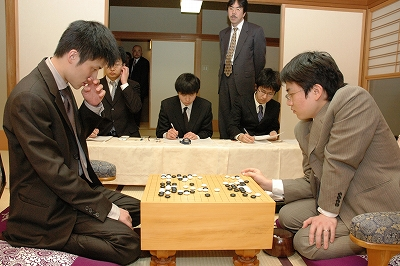Here is the game record: Cho U played black and won by resignation.

(; KM[6.5] EV[48th Judan title match 1] FF SZ GM PW[Yamashita Keigo] WR[Tengen] DT[2010-03-04] BR[Judan] RE[B+R] PB[Cho U] ;B[pd];W[dd];B[qp];W[dq];B[co];W[op];B[oq];W[nq];B[pq];W[dl];B[np] ;W[mq];B[no];W[kp];B[iq];W[fp];B[cq];W[dp];B[io];W[cp];B[lo];W[ip] ;B[jp];W[ko];B[hp];W[km];B[kq];W[lp];B[pj];W[nn];B[mo];W[ll];B[on] ;W[nc];B[lj];W[om];B[nl];W[mk];B[nm];W[mj];B[pf];W[mh];B[jd];W[hd] ;B[me];W[ce];B[jf];W[hf];B[cc];W[dc];B[hc];W[gc];B[ic];W[pb];B[qc] ;W[lc];B[gb];W[fc];B[nd];W[le];B[ld];W[kd];B[md];W[kc];B[ke];W[mc] ;B[lf];W[dj];B[cm];W[cl];B[il];W[jj];B[bp];W[bq];B[bl];W[bk];B[bn] ;W[kn];B[qb];W[ie];B[je];W[oc];B[dm];W[em];B[en];W[fm];B[fn];W[gm] ;B[br];W[cr];B[aq];W[go];B[ck];W[al];B[bm];W[dk];B[cj];W[bj];B[ci] ;W[bi];B[gn];W[hn];B[ho];W[hm];B[eo];W[gr];B[ep];W[hr];B[fq];W[fr] ;B[eq];W[er];B[ok];W[cq];B[ig];W[hh];B[ji];W[ii];B[hg];W[gg];B[ki] ;W[ih];B[jh];W[kk];B[cb];W[oi];B[pi];W[db];B[bd];W[ph];B[qh];W[be] ;B[ca];W[od];B[jb];W[kb];B[gf];W[gh];B[pc];W[fb];B[if])

### Cho U Captures Kisei Title!

Thursday, February 25th, 2010

Game five of the 34th Kisei title resulted in Cho U’s win, which ensured his capturing of the title from Yamashita Keigo by a 4-1 score. Yamashita captured the Kisei title in 2006 from Hane Naoki, then defended it for 3 consecutive years.

It looks like Cho U’s bad form from 2009 has not followed him in 2010.Here is the game record from the last game: Cho U played white and won by 1.5 points.

(;CA[Windows-1252]SZAP[MultiGo:4.4.4]GN[34th Kisei Title, game 5]EV[34th Kisei Title] DT[2010-02-26]PB[Yamashita Keigo]BR[9p]BT[Japan]PW[Cho U]WR[9p]WT[Japan]KM[6.5] HARE[W+1.5]MULTIGOGM ;B[qd];W[pp];B[dd];W[dp];B[pj];W[nd];B[oc];W[jd];B[od];W[fc];B[cf];W[qm];B[hc];W[hd] ;B[id];W[ie];B[ic];W[gd];B[jc];W[kd];B[lb];W[jf];B[cn];W[fp];B[dk];W[dg];B[fg];W[df] ;B[cg];W[de];B[ce];W[ed];B[dh];W[dc];B[cd];W[jq];B[ee];W[fe];B[ef];W[qe];B[pe];W[qf] ;B[ph];W[rd];B[qc];W[rc];B[rg];W[qg];B[qh];W[rf];B[pq];W[qq];B[oq];W[qr];B[no];W[dn] ;B[cq];W[co];B[fr];W[dr];B[bo];W[dq];B[cm];W[jo];B[lq];W[on];B[jr];W[ir];B[kr];W[hq] ;B[pg];W[op];B[np];W[pr];B[or];W[nn];B[mn];W[lo];B[mo];W[pf];B[of];W[rh];B[ri];W[sg] ;B[rl];W[rm];B[ip];W[jp];B[is];W[hs];B[hm];W[js];B[jm];W[km];B[nm];W[om];B[kn];W[jn] ;B[jl];W[ln];B[mm];W[kl];B[kk];W[lk];B[lj];W[mk];B[kj];W[nf];B[ng];W[mg];B[ne];W[mf] ;B[me];W[nh];B[og];W[le];B[ll];W[lm];B[ml];W[md];B[oe];W[lc];B[mb];W[nk];B[lp];W[ko] ;B[ol];W[mi];B[lh];W[li];B[ki];W[kh];B[jh];W[kg];B[cr];W[el];B[dl];W[gl];B[hl];W[gk] ;B[hi];W[mr];B[mq];W[os];B[ns];W[nr];B[nq];W[ps];B[nl];W[ql];B[ok];W[cc];B[eb];W[ec] ;B[em];W[fn];B[ek];W[gm];B[ho];W[hn];B[in];W[gn];B[io];W[iq];B[ds];W[hk];B[ik];W[im] ;B[il];W[kb];B[kc];W[hb];B[gb];W[ib];B[jb];W[gc];B[ka];W[fb];B[er];W[ij];B[hj];W[bd] ;B[be];W[qj];B[qi];W[bc];B[rb];W[sb];B[go];W[fo];B[gj];W[cp];B[bp];W[gg];B[gh];W[ff] ;B[eg];W[hg];B[qk];W[rk];B[rj];W[sl];B[ii];W[qb];B[pb];W[ra];B[en];W[gp];B[eo];W[fq] ;B[jj];W[gr];B[jg];W[ig];B[lg];W[kf];B[nj];W[mj];B[ni];W[mh];B[oh];W[lf];B[pm];W[pn] ;B[pl];W[fk];B[fj];W[do];B[si];W[sk];B[sh];W[rg];B[oo];W[po];B[ia];W[ga];B[pa];W[fl] ;B[dm];W[nc];B[nb];W[ae];B[af];W[ad];B[qa];W[rb];B[ih])

More photos here. You can also see a couple of videos from the previous game here.

### Yamashita’s First Win in Kisei Title Defense

Friday, February 19th, 2010

Yamashita Keigo won for the first time in this year’s Kisei title match, trying to defend the title against Cho U. The score is now 3-1 for Cho U, who has 3 more chances to capture the title from Yamashita.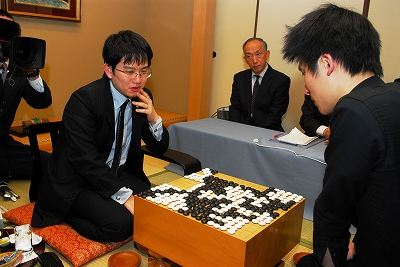Here is the record of the forth game. Yamashita Keigo, playing white, won by 7.5 points.

(;GMFFCA[UTF-8]AP[CGoban:3]ST RU[Japanese]SZKM[6.50] GN[Kisei Title 2010, game 4]PW[Yamashita Keigo]PB[Cho U]WR[9p]BR[9p]DT[2010-02-19]EV[Kisei Title 2010]RE[W+7.50]MULTIGOGM ;B[pd] ;W[dp] ;B[qp] ;W[dd] ;B[fq] ;W[cn] ;B[lq] (;W[pm] ;B[pk] ;W[op] (;B[qn] ;W[mp]LB[qm:A][pn:B]C[Since A and B are miai for black, white decided to change the course and possibly decide between A and B later, depending on black's response on the lower side.] ;B[lp] ;W[lo] ;B[mq] (;W[qq] (;B[rq] ;W[ko] (;B[iq] (;W[pq] ;B[pn] ;W[rr] ;B[om] ;W[nc] ;B[np] ;W[no] ;B[mo] ;W[mn] ;B[oo] ;W[mp] ;B[nq] ;W[rp] ;B[ro] ;W[sq] ;B[lc] ;W[ld] ;B[kd] ;W[md] ;B[jc] ;W[pf] ;B[of] ;W[qc] ;B[qd] ;W[pc] ;B[od] ;W[oc] ;B[pg] ;W[gc] ;B[kf] ;W[ce] ;B[mf] ;W[lk] ;B[dm] ;W[cm] ;B[dk] ;W[gp] ;B[dq] ;W[cq] ;B[cp] ;W[do] ;B[cr] ;W[bq] ;B[er] ;W[br] ;B[dh] ;W[lg] ;B[lf] ;W[je] ;B[he] ;W[hd] ;B[ge] ;W[ke] ;B[ie] ;W[id] ;B[jd] ;W[jf] ;B[fc] ;W[gd] ;B[fd] ;W[fb] ;B[jg] ;W[ig] ;B[fg] ;W[ih] ;B[ec] ;W[hb] ;B[dc] ;W[cc] ;B[cb] ;W[ff] ;B[gf] ;W[jb] ;B[bc] ;W[bd] ;B[cd] ;W[hq] ;B[ip] ;W[ir] ;B[jr] ;W[gr] ;B[is] ;W[hr] ;B[eo] ;W[go] ;B[fo] ;W[hn] ;B[in] ;W[im] ;B[jn] ;W[jm] ;B[kn] ;W[km] ;B[jp] ;W[fm] ;B[fn] ;W[gm] ;B[dn] ;W[co] ;B[cl] ;W[cc] ;B[bm] ;W[df] ;B[fe] ;W[ep] ;B[fp] ;W[eq] ;B[if] ;W[le] ;B[kg] ;W[ch] ;B[ci] ;W[bi] ;B[di] ;W[bg] ;B[bj] ;W[dg] ;B[ai] ;W[cd] ;B[lm] ;W[gh] ;B[fh] ;W[el] ;B[dl] ;W[nj] ;B[nl] ;W[ll] ;B[ji] ;W[ki] ;B[kj] ;W[lj] ;B[li] ;W[mi] ;B[kh] ;W[ij] ;B[oi] ;W[fi] ;B[fj] ;W[mm] ;B[ln] ;W[ml] ;B[hl] ;W[hk] ;B[il] ;W[jl] ;B[kk] ;W[kl] ;B[gk] ;W[gl] ;B[ik] ;W[jk] ;B[hj] ;W[hm] ;B[hk] ;W[fr] ;B[bo] ;W[bp] ;B[bn] ;W[dr] ;B[rc] ;W[rb] ;B[rd] ;W[ii] ;B[gi] ;W[hi] ;B[ei] ;W[fk] ;B[gj] ;W[jj] ;B[mh] ;W[or] ;B[mo] ;W[ej] ;B[fi] ;W[mp] ;B[nr] ;W[ni] ;B[nh] ;W[kp] ;B[kq] ;W[pp] ;B[mo] ;W[oj] ;B[pj] ;W[mp] ;B[ic] ;W[ib] ;B[mo] ;W[pi] ;B[oh] ;W[mp] ;B[oq] ;W[so] ;B[sn] ;W[pr] ;B[mo] ;W[qo] ;B[rn] ;W[mp] ;B[nd] ;W[mc] ;B[mo] ;W[ef] ;B[ab] ;W[ba] ;B[gg] ;W[mp] ;B[bb] ;W[mo] ;B[lr] ;W[eb] ;B[db] ;W[bh] ;B[sb] ;W[ra] ;B[ek] ;W[ah] ;B[aj] ;W[nk] ;B[bf] ;W[ad] ;B[af] ;W[ee] ;B[cf] ;W[cg] ;B[ed] ;W[ol] ;B[pl] ;W[nm] ;B[on] ;W[be] ;B[ns] ;W[ki] ;B[kj] ;W[ao] ;B[eg] ;W[ae] ;B[os] ;W[hs] ;B[js] ;W[me] ;B[ne] ;W[an] ;B[bl] ;W[da] ;B[ca] ;W[ea] ;B[ps] ;W[qs] ;B[am] ;W[ap] ;B[hg] ;W[hh] ;B[ok] ;W[po] ;B[fl] ;W[em] ;B[en] ;W[nl] ;B[fk] ;W[sp]) (;W[qm] ;B[pn] (;W[om] ;B[on] ;W[nm] ;B[nn] ;W[mn] ;B[no]) (;W[on] ;B[om] ;W[ol] ;B[nm] ;W[pl] ;B[nn])) (;W[nc] ;B[pf] ;W[jd] ;B[on] ;W[mo] ;B[om])) (;B[pn] ;W[on] (;B[np] ;W[no] ;B[mo] ;W[mn] ;B[oq] ;W[mp] ;B[nq] ;W[qm]) (;B[om] ;W[jq] ;B[np] ;W[mo] ;B[oq] ;W[no] ;B[nq] ;W[ol] ;B[nm] ;W[pl])) (;B[on] ;W[pn] ;B[po] ;W[oo] ;B[om] ;W[jq] ;B[pq] ;W[oq] ;B[qr] ;W[or] ;B[jr] ;W[ir] ;B[iq] ;W[ip] ;B[hq] ;W[kr] ;B[jp] ;W[kq] ;B[hr] ;W[kp] ;B[dr])) (;B[ko] (;W[pp] ;B[ro] ;W[pn] ;B[ql] ;W[kn] ;B[jo] ;W[jn] ;B[io] ;W[mo]) (;W[qm]))) (;W[mo]) (;W[ko] (;B[np] ;W[no] ;B[mo] ;W[mn] (;B[oq] ;W[mp] ;B[nq] ;W[qm]) (;B[pn] (;W[on] ;B[oq] ;W[mp] ;B[nq]) (;W[nq] ;B[mp] ;W[on]))) (;B[pn] ;W[on] ;B[np] ;W[oq]))) (;B[oo] ;W[no] ;B[po] ;W[nn] ;B[np] ;W[mp] ;B[nq] ;W[mq]LB[lq:A]C[Black's stone A would be hurt badly this way.])) (;W[ip]C[Also possible.]))

Next game will be played on February 25th and 26th. You can find the complete tournament table here.

### Cho U Leads 3-0 in Kisei Title

Friday, February 5th, 2010

Cho U seems to be back in good shape after a bad year: he is just one win away from capturing the Kisei title from Yamashita Keigo.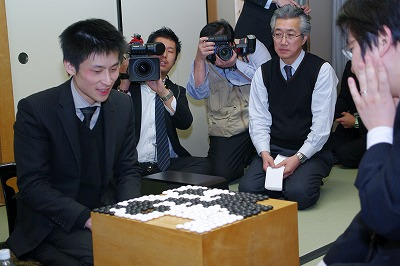Here is the record of the third game. Cho U, white, won by 8.5 points after a very confusing multi-step ko fight involving a large black group.

(;GMFFCA[UTF-8]AP[CGoban:3]ST RU[Japanese]SZKM[6.50] PW[Cho U]PB[Yamashita Keigo]WR[9p]BR[9p]DT[2010-02-03,04]EV[34th Kisei title match 3]RE[W+8.50] ;B[pd] ;W[dp] (;B[qp] (;W[ed] (;B[op] ;W[cd] (;B[pj] (;W[nc] ;B[pf] (;W[kd] ;B[dj] (;W[cl] (;B[jp] (;W[ek] (;B[fi] (;W[hj] ;B[df] ;W[ej] ;B[ei] ;W[di] ;B[dh] ;W[ci] ;B[fe] ;W[fd] ;B[ge] ;W[gd] ;B[he] ;W[hd] ;B[gk] ;W[hk] ;B[gl] ;W[hl] ;B[el] ;W[dk] ;B[gj] ;W[hn] ;B[ch] ;W[bi] ;B[je] ;W[jd] ;B[fp] ;W[hp] ;B[en] ;W[lp] ;B[ko] ;W[np] ;B[nq] ;W[oo] ;B[pp] ;W[mq] ;B[no] ;W[mo] ;B[mp] ;W[gm] ;B[fm] ;W[np] ;B[nn] ;W[nr] ;B[mp] ;W[gi] ;B[np] ;W[fj] ;B[gh] ;W[hi] ;B[cn] ;W[bp] ;B[dq] ;W[cq] ;B[eq] ;W[kq] ;B[co] ;W[cp] ;B[gn] ;W[hm] ;B[dr] ;W[cr] ;B[go] ;W[ho] ;B[bo] ;W[gp] ;B[gq] ;W[fr] ;B[fq] ;W[hq] ;B[gr] ;W[fo] ;B[eo] ;W[fn] ;B[ep] ;W[bm] ;B[ao] ;W[lo] ;B[mr] ;W[lr] ;B[lq] ;W[br] ;B[aq] ;W[mq] ;B[ce] ;W[bd] ;B[lq] ;W[hg] ;B[gg] ;W[hh] ;B[ke] ;W[le] ;B[lf] ;W[mf] ;B[lg] ;W[mg] ;B[ie] ;W[lh] ;B[kh] ;W[me] ;B[oc] ;W[nb] ;B[mq] ;W[qh] ;B[qj] ;W[pg] ;B[nd] ;W[qf] ;B[qe] ;W[rf] ;B[lc] ;W[lb] ;B[md] ;W[kc] ;B[of] ;W[ld] ;B[re] ;W[pb] ;B[qc] ;W[ne] ;B[kp] ;W[qb] ;B[rb] ;W[ra] ;B[oe] ;W[rc] ;B[rd] ;W[sb] ;B[pc] ;W[hr] ;B[jr] ;W[ap] ;B[cs] ;W[bs] ;B[ar] ;W[ds] ;B[gs] ;W[jq] ;B[kr] ;W[hs] ;B[es] ;W[dm] ;B[em] ;W[fl] ;B[jg] ;W[nh] ;B[mk] ;W[cm] ;B[cs] ;W[gf] ;B[eh] ;W[ds] ;B[do] ;W[bn] ;B[cs] ;W[nj] ;B[nk] ;W[ds] ;B[bq] ;W[an] ;B[cs] ;W[oj] ;B[ok] ;W[ds] ;B[fk] ;W[fl] ;B[cs] ;W[fg] ;B[fh] ;W[ds] ;B[fk] ;W[gk] ;B[cs] ;W[rj] ;B[rk] ;W[ds] ;B[dd] ;W[dl] ;B[cs] ;W[ee] ;B[ef] ;W[ds] ;B[go] ;W[gn] ;B[cs] ;W[hf] ;B[ff] ;W[ds] ;B[dc] ;W[dn] ;B[cs] ;W[as] ;B[aq] ;W[er] ;B[bq] ;W[ds] ;B[fs] ;W[er] ;B[cs] ;W[ar] ;B[id] ;W[ic] ;B[hc] ;W[hb] ;B[gc] ;W[fc] ;B[bq] ;W[ds] ;B[gb] ;W[fr] ;B[fb] ;W[be] ;B[bf] ;W[ae] ;B[cc] ;W[bc] ;B[bb] ;W[af] ;B[bg] ;W[ag] ;B[ah] ;W[bh] ;B[ac] ;W[ai] ;B[pi] ;W[ri] ;B[og] ;W[ph] ;B[jl] ;W[sk] ;B[rl] ;W[ib] ;B[iq] ;W[jn] ;B[kn] ;W[jm] ;B[kl] ;W[eb] ;B[ec] ;W[ga] ;B[de] ;W[fa] ;B[db] ;W[ea] ;B[sl] ;W[oh] ;B[li] ;W[km] ;B[lm] ;W[mc] ;B[mh] ;W[sf] ;B[ij] ;W[ji] ;B[jj] ;W[ii] ;B[ob] ;W[oa] ;B[ki] ;W[il] ;B[sj] ;W[od] ;B[nd] ;W[mj] ;B[lj] ;W[jk] ;B[kk] ;W[ik] ;B[si] ;W[sh] ;B[sk] ;W[ng] ;B[da] ;W[ha] ;B[qi] ;W[rh] ;B[kj] ;W[if] ;B[jf] ;W[js] ;B[ks] ;W[ip] ;B[ir] ;W[jo] ;B[oi] ;W[ni] ;B[is] ;W[mi] ;B[lh]) (;W[dh] (;B[di] ;W[eh] (;B[fk] ;W[fl] ;B[gk] ;W[gl] ;B[hk] ;W[hl] ;B[ik] ;W[jm]) (;B[fj] ;W[gl]) (;B[dk] ;W[dl] ;B[el] ;W[fk] ;B[fj] ;W[em] ;B[fl] ;W[gk])) (;B[ej] ;W[fk])) (;W[gk] ;B[hi] ;W[dh] ;B[ej]) (;W[gj] ;B[gi] ;W[hj] ;B[hi] ;W[ij] ;B[jh] ;W[kj] ;B[id])) (;B[dg] ;W[ef] ;B[fi]) (;B[ej] ;W[fk] ;B[fj] ;W[gk])) (;W[fq]) (;W[dh] ;B[ek] ;W[dn])) (;B[dg] ;W[jp]) (;B[ek] ;W[jq])) (;W[jq] ;B[cn] ;W[fp])) (;W[pb]) (;W[jp]) (;W[jc])) (;W[dj]) (;W[cj])) (;B[cn] ;W[ck])) (;B[cd] (;W[cc]) (;W[df])) (;B[cc])) (;W[dc] ;B[fq] ;W[cn] ;B[lq] ;W[de] ;B[pj] ;W[nd] ;B[lc] ;W[me] ;B[qf]) (;W[dd] ;B[op]) (;W[op])) (;B[pq] ;W[dd] ;B[fq] ;W[ip] ;B[dn] ;W[fp] ;B[cq] ;W[eq] ;B[cp] ;W[cl] ;B[bn] ;W[gq]))

Next game will be played on February 18th and 19th.

The complete tournament table is here.

### Kisei Title Started: Cho U Won Game One

Friday, January 15th, 2010

Cho U, challenger in this year’s Kisei title match, won game one against title holder Yamashita Keigo.

In their most recent encounter, Yamashita captured the Tengen title from Cho U last month.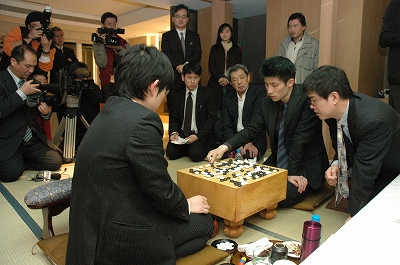The record of game one is here. Cho U, playing white, won by resignation.

(;GMFFCA[UTF-8]AP[CGoban:3]ST RU[Japanese]SZKM[6.50] GN[34th Kisei Title Match, game 1]PW[Cho U]PB[Yamashita Keigo]WR[9p]BR[9p]DT[2010-01-14,15]EV[34th Kisei Title Match]RE[W+Resign]MULTIGOGM ;B[qd] ;W[pp] ;B[dd] (;W[dp] (;B[qn] (;W[pk] (;B[np] ;W[pn] (;B[qq] (;W[qo] ;B[pq] (;W[lq] (;B[nr] (;W[qm] (;B[fq] (;W[cn] (;B[dr] (;W[gq] (;B[gp] ;W[hq] (;B[hp] (;W[fr] (;B[eq] (;W[ip] (;B[fo] (;W[ck] (;B[oc] (;W[mq] (;B[iq] ;W[ir] ;B[jq] ;W[jp] ;B[nq] ;W[jr] ;B[cq] ;W[rp] ;B[rq] ;W[cp] ;B[er] ;W[fc] ;B[hc] ;W[df] ;B[ec] ;W[fd] ;B[ee] ;W[eb] ;B[db] ;W[ed] ;B[de] ;W[dc] ;B[cc] ;W[ge] ;B[jd] ;W[qf] ;B[oe] ;W[cf] ;B[ef] ;W[dh] ;B[eh] ;W[di] ;B[ec] ;W[gg] ;B[pg] ;W[rd] ;B[rc] ;W[dc] ;B[ei] ;W[gi] ;B[ej] ;W[pd] ;B[qc] ;W[qh] ;B[dk] ;W[cl] ;B[ig] ;W[hg] ;B[eg] ;W[if] ;B[jf] ;W[jg] ;B[ih] ;W[je] ;B[ie] ;W[kf] ;B[hf] ;W[jf] ;B[he] ;W[kd] ;B[gf] ;W[jc] ;B[id] ;W[hb] ;B[ic] ;W[ib] ;B[kc] ;W[jb] ;B[ld] ;W[ke] ;B[lc] ;W[gc] ;B[fg] ;W[jh] ;B[ii] ;W[ji] ;B[jj] ;W[kj] ;B[ij] ;W[kk] ;B[li] ;W[ki] ;B[qg] ;W[rg] ;B[rh] ;W[rf] ;B[ec] ;W[ga] ;B[ri] ;W[pf] ;B[pi] ;W[qj] ;B[qi] ;W[og] ;B[ph] ;W[of] ;B[ni] ;W[od] ;B[nd] ;W[ne] ;B[pc] ;W[oh] ;B[ok] ;W[oi] ;B[pj] ;W[oj] (;B[qk] ;W[pl] ;B[rl] ;W[md] ;B[nc] ;W[il] ;B[me] ;W[nf] ;B[nn] ;W[nm] ;B[jm] ;W[hk] ;B[cj] ;W[bi] ;B[jl] ;W[jk] ;B[ik] ;W[im] ;B[in] ;W[jn] ;B[kn] ;W[jo] ;B[mm] ;W[nl] ;B[ll] ;W[hh] ;B[fk] ;W[gl] ;B[bj] ;W[dj] ;B[ci] ;W[ch] ;B[bh] ;W[dl] ;B[ek] ;W[bk] ;B[ai] ;W[mo] ;B[no] ;W[mn] ;B[mp] ;W[lo] ;B[bq] ;W[bp] ;B[ap] ;W[ao] ;B[aq] ;W[bg] ;B[dg] ;W[cg] ;B[ce] ;W[af] ;B[ah] ;W[al] ;B[bo] ;W[aj] ;B[ak] ;W[sq] ;B[sr] ;W[aj] ;B[om] ;W[ol] ;B[ak] ;W[rk] ;B[rj] ;W[aj] ;B[ea] ;W[fb] ;B[ak] ;W[sp] ;B[lp] ;W[ko] ;B[rr] ;W[aj] ;B[hm] ;W[hl] ;B[ak] ;W[an] ;B[bn] ;W[aj] ;B[kq] ;W[kp] ;B[ak] ;W[am] ;B[bm] ;W[aj] ;B[kr] ;W[lr] ;B[ak] ;W[bl] ;B[em] ;W[aj] ;B[ks] ;W[ls] ;B[ak] ;W[dn] ;B[eo] ;W[aj] ;B[da] ;W[bi]C[Black resigned.]) (;B[pl] ;W[qk] ;B[ql] ;W[ol] ;B[rk] ;W[nk] ;B[rj])) (;B[nq] ;W[cq]) (;B[cq])) (;W[cq] ;B[iq] ;W[ir] ;B[jq] ;W[jr] ;B[jp] ;W[io] ;B[jo] ;W[in] ;B[jn] ;W[im] ;B[kr])) (;B[iq] ;W[ir] ;B[jq] ;W[jr] ;B[jp] ;W[mq] (;B[io] ;W[nq] ;B[oq] ;W[oc]) (;B[nq] ;W[io] ;B[jo] ;W[in] ;B[lo] ;W[ls])) (;B[jp] (;W[io] ;B[iq] ;W[ir] ;B[jq] ;W[jr] ;B[jo] ;W[in] ;B[jn] ;W[im] ;B[kr]) (;W[iq] ;B[io] ;W[jo] ;B[kp] ;W[kr] ;B[jn] ;W[ko] ;B[lp]))) (;W[io] ;B[cq] ;W[ck]) (;W[mq] ;B[nq] ;W[cq] ;B[iq] ;W[ir] ;B[jq] ;W[jr] ;B[kq] ;W[kr] ;B[io] (;W[jp] ;B[kp] ;W[jo] ;B[lp] ;W[in] ;B[mp] ;W[ho] ;B[fm] ;W[dk] ;B[lr] ;W[er] ;B[dq] ;W[gs] ;B[hm] ;W[jl]) (;W[ck]))) (;B[cl] ;W[dl] ;B[dk] ;W[dm] (;B[fo] ;W[ck] ;B[cj] ;W[bk] ;B[dj] ;W[bl] ;B[kc]) (;B[cq] ;W[fp] ;B[ek] ;W[er] ;B[dq] ;W[fo])) (;B[iq] ;W[ir] ;B[jq] ;W[jr] ;B[kq] (;W[kr] ;B[io] ;W[jp] ;B[kp] ;W[jo] ;B[lp] ;W[fp]) (;W[io] ;B[kr] ;W[fp] ;B[hn] ;W[ep])) (;B[io] ;W[jp] ;B[fo] ;W[ck])) (;W[iq] ;B[cq] ;W[ck])) (;B[iq] ;W[ir] ;B[jr] ;W[ip] ;B[jq] ;W[eq] ;B[fp] ;W[er])) (;W[iq])) (;B[fp] ;W[cq]) (;B[cq] ;W[fr] ;B[eq] ;W[hp] ;B[fo] ;W[ck]) (;B[fr] ;W[cq] ;B[hp] ;W[iq] ;B[ep] (;W[jo]) (;W[oc] ;B[jo])) (;B[ep] ;W[dq] ;B[cr] (;W[bq] ;B[eq] ;W[hp] ;B[gn] ;W[ck]) (;W[eo] ;B[eq] ;W[fo] ;B[cp] ;W[do] ;B[bo]))) (;B[gr] ;W[fr] (;B[gp] ;W[hq] ;B[hr] ;W[eq] ;B[fp] ;W[er] ;B[iq]) (;B[er] ;W[fp] ;B[fs] ;W[hq]))) (;W[cq] ;B[iq]) (;W[hq] ;B[cq] ;W[eq] ;B[er] ;W[fp] ;B[gp] ;W[gq] ;B[fr] ;W[go] ;B[ci])) (;B[iq]LB[dr:A])) (;W[hp]) (;W[eq] ;B[fp] ;W[dn] ;B[ip] ;W[ko])) (;B[oc] (;W[fq] ;B[cf]) (;W[cf] ;B[fc] ;W[bd])) (;B[lc])) (;W[rp]) (;W[od] (;B[oc] ;W[pd] ;B[pc] ;W[qe] ;B[nc] ;W[rd] ;B[qc] ;W[pf] ;B[qm] ;W[pm] ;B[ro] ;W[ql] ;B[qp]) (;B[pf] ;W[qc] ;B[rc] ;W[pc] ;B[re] ;W[lc] ;B[qm] ;W[pm] ;B[ql]))) (;B[mq] ;W[lp] ;B[oc] ;W[no]) (;B[qm] (;W[pm] ;B[ro] ;W[ql] ;B[qp]C[Too much to expect for black.]) (;W[rp] ;B[pm] ;W[on] ;B[om] ;W[nn] ;B[nm] ;W[mn] ;B[mq] ;W[lp] ;B[rq] ;W[nk]))) (;W[od] ;B[oc] ;W[pd] ;B[pc] ;W[qe] ;B[nc] (;W[rd] ;B[qc] ;W[pf]) (;W[nd] ;B[md] ;W[qc] ;B[rd] ;W[qb] ;B[rc])) (;W[qm] ;B[oc] (;W[nq] ;B[oq] ;W[mq] ;B[op] ;W[mp]) (;W[oq] ;B[nq]) (;W[lq] ;B[mq] ;W[lp]))) (;W[pq] ;B[pm] ;W[po] ;B[pl] (;W[ok] ;B[nm] ;W[rp]) (;W[qk] (;B[ro] ;W[rq] ;B[nm] ;W[qg]) (;B[ph])))) (;B[pm] ;W[on] ;B[qo] ;W[op] ;B[rq] ;W[om])) (;B[on] ;W[np] ;B[pi]) (;B[qq] ;W[qp] ;B[pq] ;W[op] ;B[rp] ;W[ro] ;B[rq] ;W[qo] ;B[nq]) (;B[pi] ;W[np] ;B[kd]) (;B[fq] ;W[cn] ;B[np] ;W[pn] ;B[pm] ;W[on] ;B[qp] ;W[qo] ;B[ro] ;W[po] ;B[rm] ;W[qq] ;B[rp] ;W[om] ;B[jq])) (;W[nq] (;B[pj]) (;B[kd] (;W[qo] ;B[pn] ;W[pj] ;B[ph] ;W[nj] ;B[mn]) (;W[pf] (;B[od] ;W[qe] ;B[rd] ;W[qj]) (;B[ph] ;W[pd] ;B[pe] ;W[oe] ;B[qe] ;W[qc] ;B[of] ;W[pc] ;B[qf] ;W[nf] ;B[pg] ;W[nd]))) (;B[od])) (;W[np])) (;B[kc])) (;W[cp] ;B[ep] ;W[gq] ;B[cq] ;W[cm] ;B[dn] ;W[bo] ;B[fq] ;W[gp] ;B[bq] ;W[cj] ;B[oc] ;W[qn] ;B[pj] ;W[fc] ;B[hc] ;W[df] ;B[fd] ;W[cc] ;B[cd] ;W[dc] ;B[ed] ;W[fb] ;B[gc] ;W[bc] ;B[nf] ;W[gd]))

The tournament table is here. Game two will be played on January 27 and 28.

### Yamashita Keigo Captured Tengen Title from Cho U

Tuesday, December 22nd, 2009

Yamashita Keigo won the fifth game in the best-of-five Tengen title match and captured the title from Cho U with a 3:2 score.This title match must have been very tensed especially since these two will meet again very soon in an even more important battle: the first game of the Kisei title starts on January 14th with Cho as challenger, and Yamashita as the Kisei title holder.

Cho U had mixed results this year: he lost the Meijin and Tengen titles, but defended Gosei and Oza. Will he be able to capture Kisei for the first time, especially from Yamashita who has been Kisei for four consecutive years?

The 5th and decisive Tengen title game record is here. Yamashita Keigo (black) won by resignation.

(;GMFFCA[UTF-8]AP[CGoban:3]ST RU[Japanese]SZKM[6.50] GN[35th Tengen Title, game 5]PW[Cho U]PB[Yamashita Keigo]WR[9p]BR[9p]DT[2009-12-21]EV[35th Tengen Title, game 5]RO[Title match]RE[B+Resign]MULTIGOGM ;B[pd] ;W[dd] ;B[qp] ;W[op] ;B[lp] ;W[qo] ;B[ro] ;W[po] ;B[rn] ;W[qq] ;B[rq] ;W[pp] ;B[rp] ;W[pl] ;B[qr] ;W[dq] ;B[do] ;W[cm] ;B[cq] ;W[gq] ;B[dp] ;W[dr] (;B[iq] ;W[bo] ;B[bp] ;W[co] ;B[cp] ;W[go] ;B[dm] ;W[cl] (;B[gm] ;W[io] ;B[kq] (;W[hm] (;B[hl] (;W[fm] (;B[fp] ;W[hq] ;B[fl] (;W[gn] ;B[gl] (;W[ln] (;B[im] ;W[ir] (;B[no] (;W[on] (;B[mm] ;W[mn] ;B[nn] ;W[nm] ;B[nl] ;W[om] ;B[np] ;W[nq] ;B[mq] ;W[ml] ;B[lm] ;W[km] ;B[ll] ;W[mk] ;B[kl] ;W[oj] ;B[cj] ;W[dj] ;B[ci] ;W[ck] ;B[em] ;W[di] ;B[cg] ;W[ch] ;B[bh] ;W[dh] ;B[dg] ;W[bi] ;B[bj] ;W[ai] ;B[jr] ;W[ip] ;B[jq] ;W[cr] ;B[er] ;W[eq] ;B[fq] ;W[fr] ;B[gr] ;W[es] ;B[hr] ;W[ep] ;B[fo] ;W[eo] ;B[fn] ;W[dn] ;B[bf] ;W[bk] ;B[cc] ;W[dc] ;B[cd] ;W[hc] ;B[lj] ;W[mj] ;B[jc] ;W[nc] ;B[oc] ;W[nd] ;B[pf] ;W[ie] ;B[ke] ;W[kj] ;B[li] ;W[lk] ;B[kk] ;W[ng] ;B[kh] ;W[kd] ;B[ld] ;W[jd] ;B[lc] ;W[le] ;B[me] ;W[lf] ;B[mf] ;W[mg] ;B[lg] ;W[kf] ;B[jf] ;W[je] ;B[kg] ;W[ke] ;B[oe] ;W[md] ;B[ne] ;W[kc] ;B[lb] ;W[mb] ;B[mi] ;W[ni] ;B[pi] ;W[jj] ;B[ik] ;W[pj] ;B[oh] ;W[ki] ;B[ig] ;W[lh] ;B[mh] ;W[nh] ;B[lh] ;W[ce] ;B[be] ;W[qh] ;B[qi] ;W[og] ;B[pg] ;W[ph] ;B[rh] ;W[qg] ;B[rg] ;W[qf] ;B[qe] ;W[ij] ;B[gg] ;W[gi] ;B[gh] ;W[jk] ;B[jl] ;W[hk] ;B[fi] ;W[il] ;B[jm] ;W[hn] ;B[ik] ;W[ih] ;B[hg] ;W[il] ;B[kn] ;W[fj] ;B[gj] ;W[gk] ;B[ej] ;W[fk] ;B[ok] ;W[ol] ;B[nk] ;W[nj] ;B[qm]) (;B[nn] ;W[np]) (;B[np] ;W[mm] ;B[oq])) (;W[nn] ;B[on] ;W[nm] ;B[oo] ;W[mo] ;B[np] ;W[mp] ;B[mq] ;W[nq] ;B[oq] ;W[nr] ;B[pn] ;W[mr] ;B[lq] ;W[js] ;B[jp])) (;B[cj] ;W[dj] ;B[ci] ;W[ck] (;B[em] ;W[di] ;B[cg] ;W[dh] ;B[bf] ;W[ce] ;B[dg] ;W[eg] ;B[ef] ;W[fg]) (;B[di] ;W[em] ;B[dl] ;W[el] ;B[dk] ;W[dn])) (;B[ip] ;W[in] ;B[jr])) (;B[dl]) (;B[dj]) (;B[cj])) (;W[em] ;B[dl] (;W[dn] ;B[en] ;W[cn] ;B[fn]LB[eo:A]SQ[fp]C[The square marked black stone protects against white's cut at A.]) (;W[el] ;B[dk] ;W[ek] ;B[ej] ;W[fk] ;B[dn] ;W[ln]))) (;W[em] ;B[dl] ;W[el] ;B[dk] ;W[fk] ;B[ck] ;W[gl])) (;B[fl] ;W[gn] ;B[gl] ;W[em] (;B[fp]) (;B[dl] ;W[dn] ;B[en] ;W[cn] ;B[fn] ;W[eo]))) (;W[im] ;B[gn] ;W[ho] ;B[lm]) (;W[gl] ;B[fm] (;W[hk] ;B[il] ;W[im] ;B[dl]) (;W[en] ;B[dn] ;W[em]))) (;B[gl] ;W[ln])) (;W[hq]) (;W[jr]) (;W[ek] ;B[im])) (;B[fm]) (;B[dl])) (;B[go]))

The complete tournament table is here.

### Yamashita Keigo – Cho U 2:1 in Tengen

Wednesday, December 2nd, 2009

Yamashita Keigo won the third game from the Tengen title match, and leads title-holder Cho U 2:1.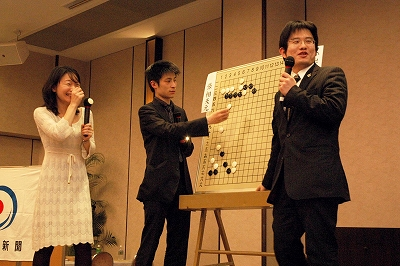The game record is here. Yamashita Keigo (black) won by resignation.

(;CA[Windows-1252]SZAP[MultiGo:4.4.4]GN[Tengen Title 2009]EV[Tengen Title 2009] DT[2009-12-02]PB[Yamashita Keigo]PW[Cho U]KM[6.5]HARE[B+R]MULTIGOGM ;B[qd];W[pp];B[dd];W[cp];B[ep];W[gq];B[cq];W[cm];B[dn];W[bo];B[fq];W[gp];B[bq];W[cj] ;B[oc];W[qn];B[pj];W[fc];B[hc];W[df];B[fd];W[cc];B[cd];W[dc];B[ed];W[fb];B[gc];W[bc] ;B[nf];W[gd];B[id];W[ge];B[ef];W[gg];B[eh];W[gi];B[ej];W[di];B[fh];W[gh];B[fj];W[hk] ;B[dm];W[cl];B[dk];W[je];B[ie];W[jg];B[if];W[kh];B[ff];W[nh];B[ph];W[og];B[nj];W[pg] ;B[qg];W[qf];B[qh];W[pe];B[nd];W[re];B[rd];W[me];B[ne];W[mi];B[cn];W[dl];B[bn];W[el] ;B[gf];W[qk];B[mj];W[kk];B[kj];W[pd];B[pc];W[rc];B[qc];W[lj];B[pk];W[lk];B[ql];W[oq] ;B[kq];W[iq];B[mq];W[mo];B[lo];W[ln];B[ko];W[mp];B[lp];W[rl];B[qm];W[rm];B[pn];W[qj] ;B[qo];W[rn];B[po];W[pm];B[pl];W[om];B[nn];W[nm];B[mn];W[mm];B[on];W[ri];B[lm];W[ml] ;B[ro];W[rq];B[rh];W[oi];B[pi];W[sn];B[si];W[sj];B[qi];W[sh];B[sg];W[np];B[jm];W[sf] ;B[si];W[rj];B[rp];W[sh];B[qq])

Next game will be played on December 10th. You can find the complete tournament table here.

### 11th Nongshim Cup: Kim Jiseok Eliminated Yamashita Keigo

Wednesday, November 25th, 2009

The 11th Nongshim Cup started with Kim Jiseok 6p of S. Korea eliminating Yamashita Keigo 9p (and Kisei title holder) of Japan.Here are the players from each of the three participating countries:

Korea: Lee Changho(9p), Park Yeonghun(9p), Yun Junsang(7p), Kim Jiseok(6p), Kim Seongjae(3p)

China: Chang Hao(9p), Ding Wei(9p), Gu Li(9p), Xie He(7p), Liu Xing(7p)

Japan: Yamada Kimio(9p), Hane Naoki(9p), Takao Shinji(9p), Yamashita Keigo(9p), Iyama Yuta(9p)

Next game will be played tomorrow (or tonight in the US) with Kim Jiseok being matched against one of the Chinese players.

Tournament table here.

### Cho U Evens the Score in Tengen Title Match

Thursday, November 19th, 2009

Cho U, Tengen title holder, defeated the challenger Yamashita Keigo in the second game.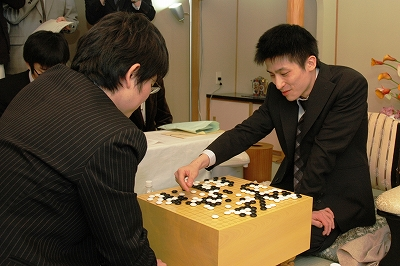The game record is here. Cho U, playing black, won by resignation.

(; KM[6.5] EV[35th Tengen title match 1] FF SZ GM PW[Yamashita Keigo] WR[Kisei] DT[2009-11-19] BR[Tengen] RE[B+R] PB[Cho U] ;B[pd];W[dp];B[qp];W[dc];B[fq];W[cn];B[lq];W[de];B[pj];W[nc];B[lc] ;W[qc];B[qd];W[pc];B[od];W[nd];B[oc];W[ob];B[pb];W[qb];B[nb];W[pa] ;B[ne];W[mb];B[me];W[ip];B[fo];W[in];B[dr];W[cq];B[fm];W[il];B[op] ;W[gp];B[ep];W[en];B[fn];W[gq];B[do];W[co];B[dq];W[cp];B[jo];W[jn] ;B[ko];W[kq];B[kr];W[jq];B[lp];W[fr];B[em];W[dn];B[eo];W[fk];B[dl] ;W[bl];B[ck];W[bk];B[gk];W[gl];B[io];W[hn];B[ho];W[go];B[gn];W[jr] ;B[er];W[gr];B[di];W[fi];B[bj];W[cg];B[lr];W[ir];B[dg];W[ch];B[dh] ;W[df];B[ci];W[fg];B[ek];W[fl];B[ej];W[fj];B[br];W[cr];B[cs];W[bq] ;B[bf];W[cf];B[bh];W[rp];B[ro];W[qo];B[po];W[qn];B[rq];W[oh];B[qh] ;W[of];B[qf];W[jd];B[mc];W[na];B[nj];W[mh];B[md];W[ql];B[qk];W[rk] ;B[rj];W[ok];B[oj];W[om];B[nl];W[pk];B[qj];W[pn];B[lj];W[oo];B[pp] ;W[mk];B[nk];W[rn];B[sp];W[sl];B[ol];W[pl];B[no];W[on];B[sn];W[rm] ;B[sj];W[sk];B[je];W[ie];B[jf];W[li];B[id];W[jc];B[jh];W[ig];B[if] ;W[he];B[hf];W[ge];B[kj];W[bg];B[ag];W[mj];B[ll];W[ki];B[ji];W[jj] ;B[kl];W[jg];B[kg];W[kf];B[hg];W[ih];B[kh];W[lf];B[hi];W[ke];B[kd] )

Next game will be played on December 3rd.